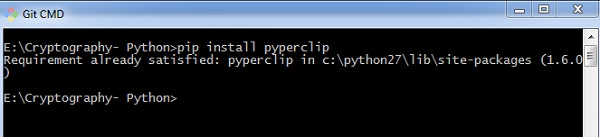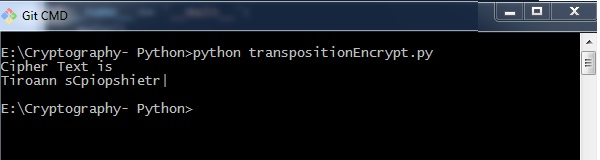# Encryption of Transposition Cipher

In the previous chapter, we have learnt about Transposition Cipher. In this chapter, let us discuss its encryption.

## Pyperclip

The main usage of pyperclip plugin in Python programming language is to perform cross platform module for copying and pasting text to the clipboard. You can install python pyperclip module using the command as shown

```>pip install pyperclip
```

If the requirement already exists in the system, you can see the following output −### Code

The python code for encrypting transposition cipher in which pyperclip is the main module is as shown below −

```import pyperclip
def main():
myMessage = 'Transposition Cipher'
myKey = 10
ciphertext = encryptMessage(myKey, myMessage)

print("Cipher Text is")
print(ciphertext + '|')
pyperclip.copy(ciphertext)

def encryptMessage(key, message):
ciphertext = [''] * key

for col in range(key):
position = col
while position < len(message):
ciphertext[col] += message[position]
position += key
return ''.join(ciphertext) #Cipher text
if __name__ == '__main__':
main()```

### Output

The program code for encrypting transposition cipher in which pyperclip is the main module gives the following output −### Explanation

• The function main() calls the encryptMessage() which includes the procedure for splitting the characters using len function and iterating them in a columnar format.

• The main function is initialized at the end to get the appropriate output.